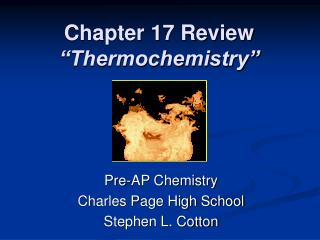DownloadDownload PresentationChapter 17 Review “Thermochemistry”

# Chapter 17 Review “Thermochemistry”

Download Presentation## Chapter 17 Review “Thermochemistry”

- - - - - - - - - - - - - - - - - - - - - - - - - - - E N D - - - - - - - - - - - - - - - - - - - - - - - - - - -
##### Presentation Transcript

1. Chapter 17 Review“Thermochemistry” Pre-AP Chemistry Charles Page High School Stephen L. Cotton

2. Chapter 17 Review • What would likely happen (how would it feel) if you were to touch the flask in which an endothermic reaction were occurring? • Standard conditions of temperature and pressure for a thermochemical equation are __ and __kPa. • What is the definition for heat of solution? oC

3. Chapter 17 Review • Hess’s law makes it possible to ___. • If heat is released by a chemical system, an equal amount of heat will be ____. • By what quantity must the heat capacity (J/oC) of an object be divided to obtain the specific heat (J/goC) of that material?

4. Chapter 17 Review • The symbol stands for the ____. • When energy is changed from one form to another, ____. • What happens to the energy produced by burning gasoline in a car engine? • How can the enthalpy change be determined for a reaction in an aqueous solution?

5. Chapter 17 Review • A process that absorbs heat is called a(n) ____ process. • What does the symbol ΔH stand for? • During a phase change, the temperature of a substance ____. • The amount of heat needed to melt one mole of a solid at a constant temperature is called ____.

6. Chapter 17 Review • Using a table that lists standard heats of formation, you can calculate the change in enthalpy for a given chemical reaction. The change is equal to ____. • A piece of metal is heated, then submerged in cool water. The temperature of the water ____ and the temperature of the metal ____.

7. Chapter 17 Review • The amount of heat released by the complete burning of 1 mole of a substance is the ____. • The ΔHsoln is a value that is ____. • In an exothermic reaction, the energy stored in the chemical bonds of the reactants is ____ than the energy stored in the bonds of the products.

8. Chapter 17 Review • The amount of heat involved in the synthesis of 1 mole of compound from its elements, with all substances in their standard states at 25 oC, is called ____. • Which of the following substances has the highest specific heat: a) alcohol, or b) water?

9. Chapter 17 Review • On what principle does calorimetry depend? • The specific heat capacity of graphite is 0.71 J/g oC. Calculate the energy required to raise the temperature of 450 g of graphite by 16 oC.

10. Chapter 17 Review • Consider a 79 g chunk of ice (ΔHfus = 6.0 kJ/mol) in a beaker immersed in a water bath. To produce just enough heat to melt the ice, how many moles of solid NaOH (ΔHsoln = -445.1 kJ/mol) must you dissolve in the water bath?

11. Chapter 17 Review • A 25.0 g piece of copper wire is heated, and the temperature of the wire changes from 29.0 oC to 86.0 oC. The amount of heat absorbed is 343 cal. What is the specific heat of copper?

12. Chapter 17 Review • Use the information below to calculate ΔHo for the reaction. Target equation: 2NO2(g) → N2O4(g) Given equations: N2(g) + 2O2(g) → 2NO2(g)ΔHo = 67.7 kJ N2(g) + 2O2(g) → N2O4(g)ΔHo = 9.7 kJ

13. Chapter 17 Review • How much heat is required to raise the temperature of 6.5 x 102 g of aluminum by 30 oC? (specific heat of aluminum = 0.21 cal/g oC) • How many joules are there in 125 calories? (1 cal = 4.184 J) • How much heat is required to melt 4.6 mol of NaCl (ΔHfus = 30.2 kJ/mol) at its melting point?

14. Chapter 17 Review • It takes 470 joules of energy to raise the temperature of 50.0 g of mercury by 90 oC. What is the specific heat of mercury? • A substance releases 296 kJ of heat as 1.60 mol condenses from a gas into a liquid. What is the molar heat of vaporization of the substance?

15. Chapter 17 Review • When 34.0 g of methanol (CH3OH) is burned, 954 kJ of energy is produced. What is the heat of combustion (in kJ/mol) for methanol?

16. Chapter 17 Review • A certain substance with a molar mass of 43 g/mol has a heat of fusion of 28 cal/g. How many calories are needed to melt 5.2 kg of the substance? • If 150 g of iron absorbs 2,000 cal of heat, what will be the change in temperature? (specific heat of iron = 0.11 cal/g oC)

17. Chapter 17 Review • If you supply 36 kJ of heat, how many moles of ice at 0 oC can be melted, heated to its boiling point, and completely boiled away? Use the following information: ΔHvap = 40.5 kJ/mol Specific heatwater = 0.0753 kJ/mol oC ΔHfus = 6.0 kJ/mol

18. Chapter 17 Review End of Chapter 17 Review